# 6.2 Graphs of the other trigonometric functions  (Page 9/9)

 Page 9 / 9

How can the graph of $\text{\hspace{0.17em}}y=\mathrm{cos}\text{\hspace{0.17em}}x\text{\hspace{0.17em}}$ be used to construct the graph of $\text{\hspace{0.17em}}y=\mathrm{sec}\text{\hspace{0.17em}}x?$

Explain why the period of $\text{\hspace{0.17em}}\mathrm{tan}\text{\hspace{0.17em}}x\text{\hspace{0.17em}}$ is equal to $\text{\hspace{0.17em}}\pi .$

Answers will vary. Using the unit circle, one can show that $\text{\hspace{0.17em}}\mathrm{tan}\left(x+\pi \right)=\mathrm{tan}\text{\hspace{0.17em}}x.\text{\hspace{0.17em}}$

Why are there no intercepts on the graph of $\text{\hspace{0.17em}}y=\mathrm{csc}\text{\hspace{0.17em}}x?$

How does the period of $\text{\hspace{0.17em}}y=\mathrm{csc}\text{\hspace{0.17em}}x\text{\hspace{0.17em}}$ compare with the period of $\text{\hspace{0.17em}}y=\mathrm{sin}\text{\hspace{0.17em}}x?$

The period is the same: $\text{\hspace{0.17em}}2\pi .$

## Algebraic

For the following exercises, match each trigonometric function with one of the following graphs.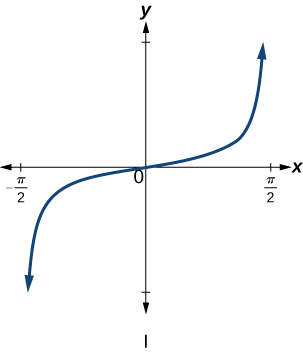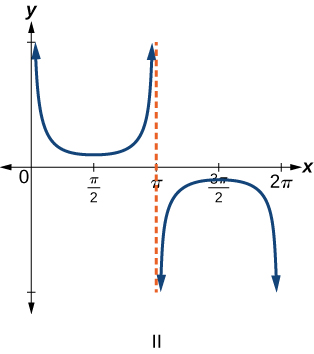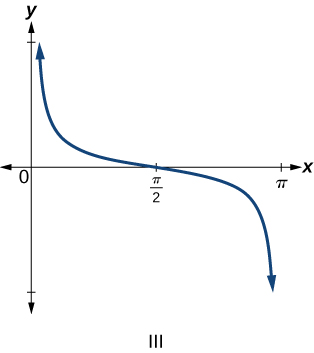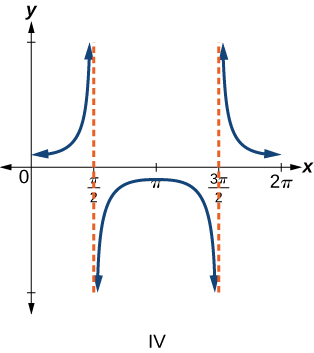$f\left(x\right)=\mathrm{tan}\text{\hspace{0.17em}}x$

$f\left(x\right)=\mathrm{sec}\text{\hspace{0.17em}}x$

IV

$f\left(x\right)=\mathrm{csc}\text{\hspace{0.17em}}x$

$f\left(x\right)=\mathrm{cot}\text{\hspace{0.17em}}x$

III

For the following exercises, find the period and horizontal shift of each of the functions.

$f\left(x\right)=2\mathrm{tan}\left(4x-32\right)$

$h\left(x\right)=2\mathrm{sec}\left(\frac{\pi }{4}\left(x+1\right)\right)$

period: 8; horizontal shift: 1 unit to left

$m\left(x\right)=6\mathrm{csc}\left(\frac{\pi }{3}x+\pi \right)$

If $\text{\hspace{0.17em}}\mathrm{tan}\text{\hspace{0.17em}}x=-1.5,\text{\hspace{0.17em}}$ find $\text{\hspace{0.17em}}\mathrm{tan}\left(-x\right).$

1.5

If $\text{\hspace{0.17em}}\mathrm{sec}\text{\hspace{0.17em}}x=2,\text{\hspace{0.17em}}$ find $\text{\hspace{0.17em}}\mathrm{sec}\left(-x\right).$

If $\text{\hspace{0.17em}}\mathrm{csc}\text{\hspace{0.17em}}x=-5,\text{\hspace{0.17em}}$ find $\text{\hspace{0.17em}}\mathrm{csc}\left(-x\right).$

5

If $\text{\hspace{0.17em}}x\mathrm{sin}\text{\hspace{0.17em}}x=2,\text{\hspace{0.17em}}$ find $\text{\hspace{0.17em}}\left(-x\right)\mathrm{sin}\left(-x\right).$

For the following exercises, rewrite each expression such that the argument $\text{\hspace{0.17em}}x\text{\hspace{0.17em}}$ is positive.

$\mathrm{cot}\left(-x\right)\mathrm{cos}\left(-x\right)+\mathrm{sin}\left(-x\right)$

$-\mathrm{cot}x\mathrm{cos}x-\mathrm{sin}x$

$\mathrm{cos}\left(-x\right)+\mathrm{tan}\left(-x\right)\mathrm{sin}\left(-x\right)$

## Graphical

For the following exercises, sketch two periods of the graph for each of the following functions. Identify the stretching factor, period, and asymptotes.

$f\left(x\right)=2\mathrm{tan}\left(4x-32\right)$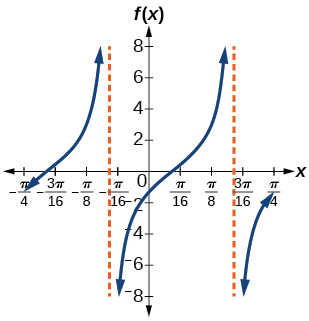stretching factor: 2; period: asymptotes:

$\text{\hspace{0.17em}}h\left(x\right)=2\mathrm{sec}\left(\frac{\pi }{4}\left(x+1\right)\right)\text{\hspace{0.17em}}$

$m\left(x\right)=6\mathrm{csc}\left(\frac{\pi }{3}x+\pi \right)$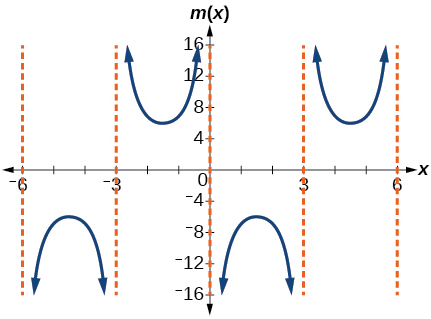stretching factor: 6; period: 6; asymptotes:

$j\left(x\right)=\mathrm{tan}\left(\frac{\pi }{2}x\right)$

$p\left(x\right)=\mathrm{tan}\left(x-\frac{\pi }{2}\right)$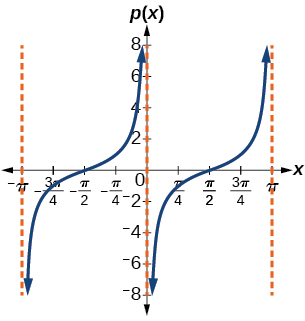stretching factor: 1; period: asymptotes:

$f\left(x\right)=4\mathrm{tan}\left(x\right)$

$f\left(x\right)=\mathrm{tan}\left(x+\frac{\pi }{4}\right)$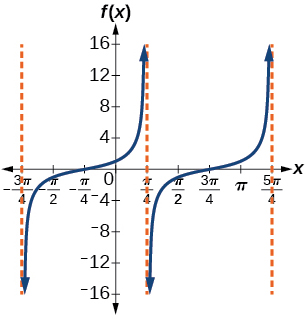Stretching factor: 1; period: asymptotes:

$f\left(x\right)=\pi \mathrm{tan}\left(\pi x-\pi \right)-\pi$

$f\left(x\right)=2\mathrm{csc}\left(x\right)$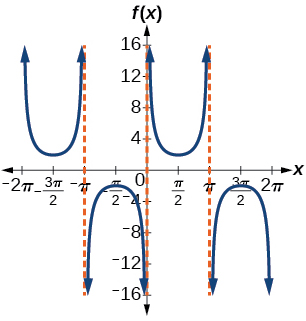stretching factor: 2; period: asymptotes:

$f\left(x\right)=-\frac{1}{4}\mathrm{csc}\left(x\right)$

$f\left(x\right)=4\mathrm{sec}\left(3x\right)$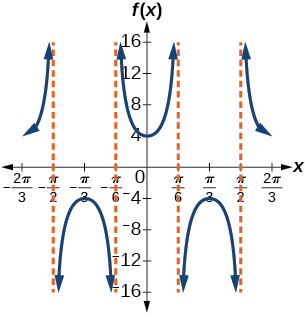stretching factor: 4; period: asymptotes:

$f\left(x\right)=-3\mathrm{cot}\left(2x\right)$

$f\left(x\right)=7\mathrm{sec}\left(5x\right)$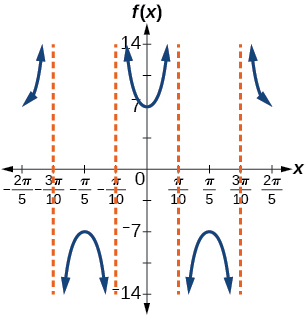stretching factor: 7; period: asymptotes:

$f\left(x\right)=\frac{9}{10}\mathrm{csc}\left(\pi x\right)$

$f\left(x\right)=2\mathrm{csc}\left(x+\frac{\pi }{4}\right)-1$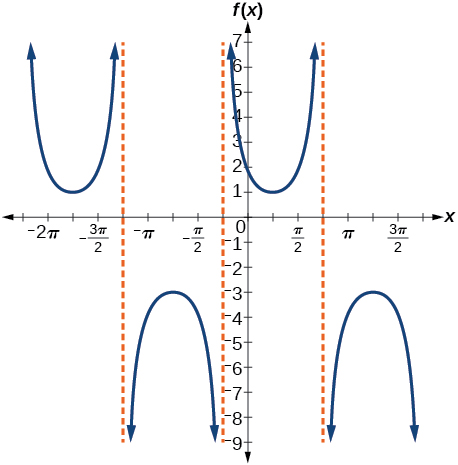stretching factor: 2; period: asymptotes:

$f\left(x\right)=-\mathrm{sec}\left(x-\frac{\pi }{3}\right)-2$

$f\left(x\right)=\frac{7}{5}\mathrm{csc}\left(x-\frac{\pi }{4}\right)$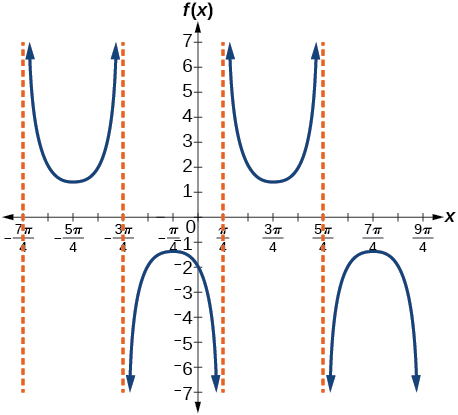stretching factor: period: asymptotes:

$f\left(x\right)=5\left(\mathrm{cot}\left(x+\frac{\pi }{2}\right)-3\right)$

For the following exercises, find and graph two periods of the periodic function with the given stretching factor, $\text{\hspace{0.17em}}|A|,\text{\hspace{0.17em}}$ period, and phase shift.

A tangent curve, $\text{\hspace{0.17em}}A=1,\text{\hspace{0.17em}}$ period of $\text{\hspace{0.17em}}\frac{\pi }{3};\text{\hspace{0.17em}}$ and phase shift $\text{\hspace{0.17em}}\left(h,\text{\hspace{0.17em}}k\right)=\left(\frac{\pi }{4},2\right)$

$y=\mathrm{tan}\left(3\left(x-\frac{\pi }{4}\right)\right)+2$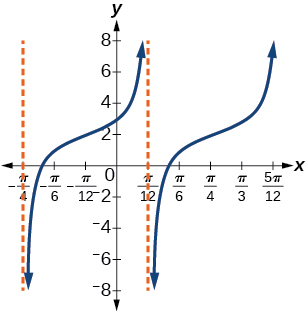A tangent curve, $\text{\hspace{0.17em}}A=-2,\text{\hspace{0.17em}}$ period of $\text{\hspace{0.17em}}\frac{\pi }{4},\text{\hspace{0.17em}}$ and phase shift $\text{\hspace{0.17em}}\left(h,\text{\hspace{0.17em}}k\right)=\left(-\frac{\pi }{4},\text{\hspace{0.17em}}-2\right)$

For the following exercises, find an equation for the graph of each function.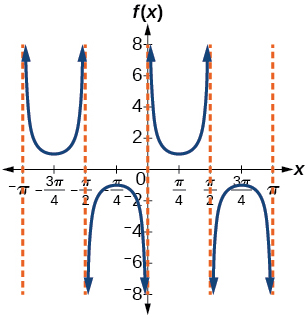$f\left(x\right)=\mathrm{csc}\left(2x\right)$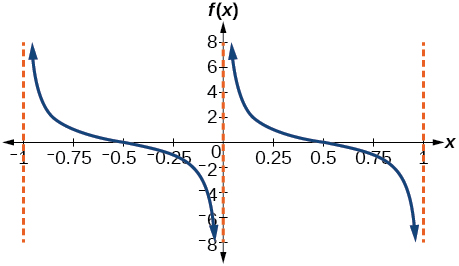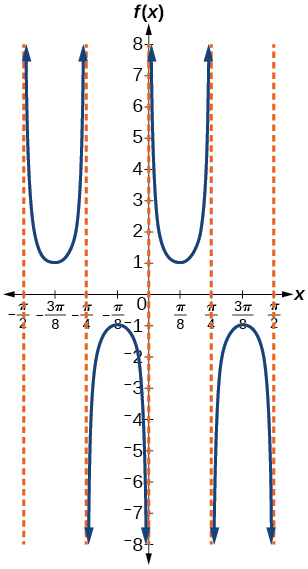$f\left(x\right)=\mathrm{csc}\left(4x\right)$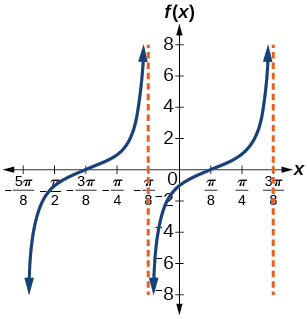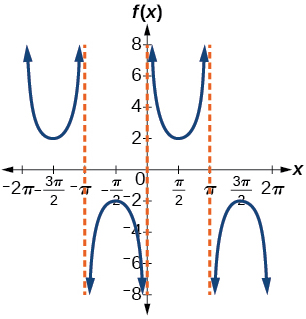$f\left(x\right)=2\mathrm{csc}x$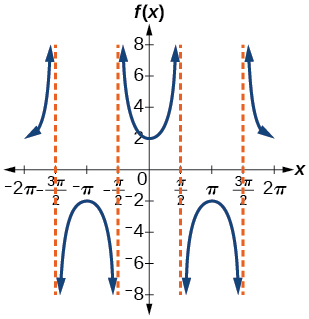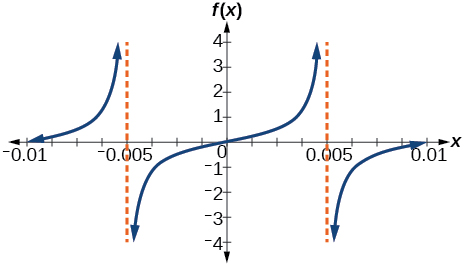$f\left(x\right)=\frac{1}{2}\mathrm{tan}\left(100\pi x\right)$

## Technology

For the following exercises, use a graphing calculator to graph two periods of the given function. Note: most graphing calculators do not have a cosecant button; therefore, you will need to input $\text{\hspace{0.17em}}\mathrm{csc}\text{\hspace{0.17em}}x\text{\hspace{0.17em}}$ as $\text{\hspace{0.17em}}\frac{1}{\mathrm{sin}\text{\hspace{0.17em}}x}.$

$f\left(x\right)=|\mathrm{csc}\left(x\right)|$

$f\left(x\right)=|\mathrm{cot}\left(x\right)|$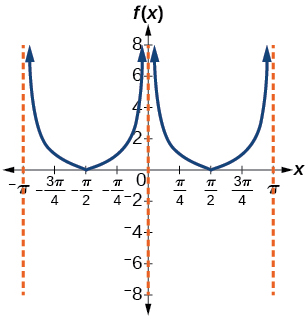$f\left(x\right)={2}^{\mathrm{csc}\left(x\right)}$

$f\left(x\right)=\frac{\mathrm{csc}\left(x\right)}{\mathrm{sec}\left(x\right)}$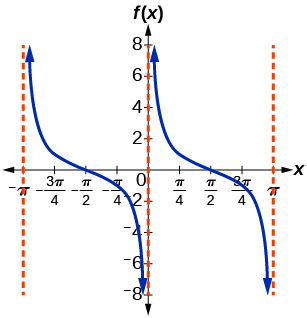Graph $\text{\hspace{0.17em}}f\left(x\right)=1+{\mathrm{sec}}^{2}\left(x\right)-{\mathrm{tan}}^{2}\left(x\right).\text{\hspace{0.17em}}$ What is the function shown in the graph?

$f\left(x\right)=\mathrm{sec}\left(0.001x\right)$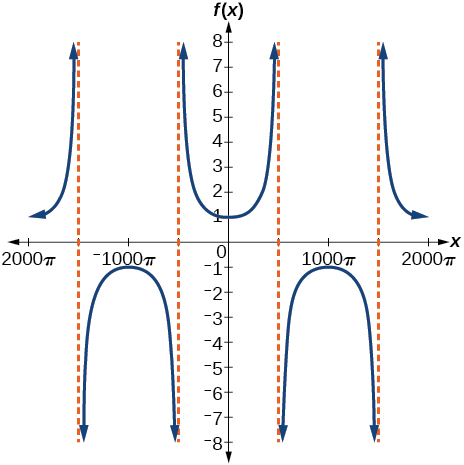$f\left(x\right)=\mathrm{cot}\left(100\pi x\right)$

$f\left(x\right)={\mathrm{sin}}^{2}x+{\mathrm{cos}}^{2}x$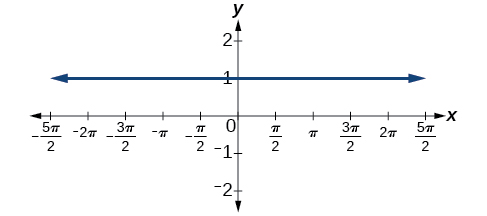## Real-world applications

The function $\text{\hspace{0.17em}}f\left(x\right)=20\mathrm{tan}\left(\frac{\pi }{10}x\right)\text{\hspace{0.17em}}$ marks the distance in the movement of a light beam from a police car across a wall for time $\text{\hspace{0.17em}}x,\text{\hspace{0.17em}}$ in seconds, and distance $\text{\hspace{0.17em}}f\left(x\right),$ in feet.

1. Graph on the interval $\text{\hspace{0.17em}}\left[0,\text{\hspace{0.17em}}5\right].$
2. Find and interpret the stretching factor, period, and asymptote.
3. Evaluate $\text{\hspace{0.17em}}f\left(1\right)\text{\hspace{0.17em}}$ and $\text{\hspace{0.17em}}f\left(2.5\right)\text{\hspace{0.17em}}$ and discuss the function’s values at those inputs.

Standing on the shore of a lake, a fisherman sights a boat far in the distance to his left. Let $\text{\hspace{0.17em}}x,\text{\hspace{0.17em}}$ measured in radians, be the angle formed by the line of sight to the ship and a line due north from his position. Assume due north is 0 and $\text{\hspace{0.17em}}x\text{\hspace{0.17em}}$ is measured negative to the left and positive to the right. (See [link] .) The boat travels from due west to due east and, ignoring the curvature of the Earth, the distance $\text{\hspace{0.17em}}d\left(x\right),\text{\hspace{0.17em}}$ in kilometers, from the fisherman to the boat is given by the function $\text{\hspace{0.17em}}d\left(x\right)=1.5\mathrm{sec}\left(x\right).$

1. What is a reasonable domain for $\text{\hspace{0.17em}}d\left(x\right)?$
2. Graph $\text{\hspace{0.17em}}d\left(x\right)\text{\hspace{0.17em}}$ on this domain.
3. Find and discuss the meaning of any vertical asymptotes on the graph of $\text{\hspace{0.17em}}d\left(x\right).$
4. Calculate and interpret $\text{\hspace{0.17em}}d\left(-\frac{\pi }{3}\right).\text{\hspace{0.17em}}$ Round to the second decimal place.
5. Calculate and interpret $\text{\hspace{0.17em}}d\left(\frac{\pi }{6}\right).\text{\hspace{0.17em}}$ Round to the second decimal place.
6. What is the minimum distance between the fisherman and the boat? When does this occur?
1. $\text{\hspace{0.17em}}\left(-\frac{\pi }{2},\text{\hspace{0.17em}}\frac{\pi }{2}\right);\text{\hspace{0.17em}}$
2.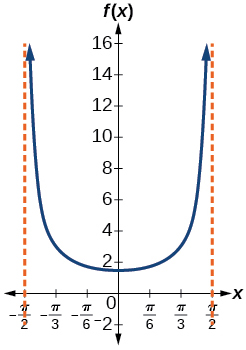3. $\text{\hspace{0.17em}}x=-\frac{\pi }{2}\text{\hspace{0.17em}}$ and $\text{\hspace{0.17em}}x=\frac{\pi }{2};\text{\hspace{0.17em}}$ the distance grows without bound as $\text{\hspace{0.17em}}|x|$ approaches $\text{\hspace{0.17em}}\frac{\pi }{2}\text{\hspace{0.17em}}$ —i.e., at right angles to the line representing due north, the boat would be so far away, the fisherman could not see it;
4. 3; when $\text{\hspace{0.17em}}x=-\frac{\pi }{3},\text{\hspace{0.17em}}$ the boat is 3 km away;
5. 1.73; when $\text{\hspace{0.17em}}x=\frac{\pi }{6},\text{\hspace{0.17em}}$ the boat is about 1.73 km away;
6. 1.5 km; when $\text{\hspace{0.17em}}x=0\text{\hspace{0.17em}}$

A laser rangefinder is locked on a comet approaching Earth. The distance $\text{\hspace{0.17em}}g\left(x\right),\text{\hspace{0.17em}}$ in kilometers, of the comet after $\text{\hspace{0.17em}}x\text{\hspace{0.17em}}$ days, for $\text{\hspace{0.17em}}x\text{\hspace{0.17em}}$ in the interval 0 to 30 days, is given by $\text{\hspace{0.17em}}g\left(x\right)=250,000\mathrm{csc}\left(\frac{\pi }{30}x\right).$

1. Graph $\text{\hspace{0.17em}}g\left(x\right)\text{\hspace{0.17em}}$ on the interval $\text{\hspace{0.17em}}\left[0,\text{\hspace{0.17em}}35\right].$
2. Evaluate $\text{\hspace{0.17em}}g\left(5\right)\text{\hspace{0.17em}}$ and interpret the information.
3. What is the minimum distance between the comet and Earth? When does this occur? To which constant in the equation does this correspond?
4. Find and discuss the meaning of any vertical asymptotes.

A video camera is focused on a rocket on a launching pad 2 miles from the camera. The angle of elevation from the ground to the rocket after $\text{\hspace{0.17em}}x\text{\hspace{0.17em}}$ seconds is $\text{\hspace{0.17em}}\frac{\pi }{120}x.$

1. Write a function expressing the altitude $\text{\hspace{0.17em}}h\left(x\right),\text{\hspace{0.17em}}$ in miles, of the rocket above the ground after $\text{\hspace{0.17em}}x\text{\hspace{0.17em}}$ seconds. Ignore the curvature of the Earth.
2. Graph $\text{\hspace{0.17em}}h\left(x\right)\text{\hspace{0.17em}}$ on the interval $\text{\hspace{0.17em}}\left(0,\text{\hspace{0.17em}}60\right).$
3. Evaluate and interpret the values $\text{\hspace{0.17em}}h\left(0\right)\text{\hspace{0.17em}}$ and $\text{\hspace{0.17em}}h\left(30\right).$
4. What happens to the values of $\text{\hspace{0.17em}}h\left(x\right)\text{\hspace{0.17em}}$ as $\text{\hspace{0.17em}}x\text{\hspace{0.17em}}$ approaches 60 seconds? Interpret the meaning of this in terms of the problem.
1. $h\left(x\right)=2\mathrm{tan}\left(\frac{\pi }{120}x\right);$
2.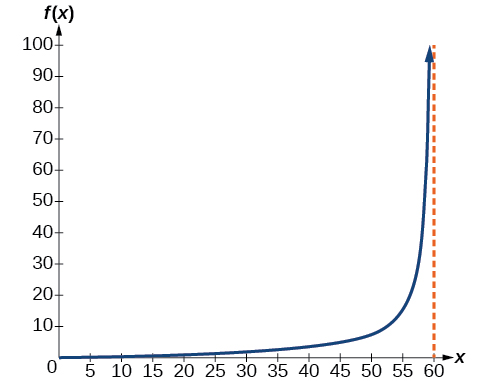3. $h\left(0\right)=0:\text{\hspace{0.17em}}$ after 0 seconds, the rocket is 0 mi above the ground; $h\left(30\right)=2:\text{\hspace{0.17em}}$ after 30 seconds, the rockets is 2 mi high;
4. As $\text{\hspace{0.17em}}x\text{\hspace{0.17em}}$ approaches 60 seconds, the values of $\text{\hspace{0.17em}}h\left(x\right)\text{\hspace{0.17em}}$ grow increasingly large. The distance to the rocket is growing so large that the camera can no longer track it.

a colony of bacteria is growing exponentially doubling in size every 100 minutes. how much minutes will it take for the colony of bacteria to triple in size
I got 300 minutes. is it right?
Patience
no. should be about 150 minutes.
Jason
It should be 158.5 minutes.
Mr
ok, thanks
Patience
what is the importance knowing the graph of circular functions?
can get some help basic precalculus
What do you need help with?
Andrew
how to convert general to standard form with not perfect trinomial
can get some help inverse function
ismail
Rectangle coordinate
how to find for x
it depends on the equation
Robert
whats a domain
The domain of a function is the set of all input on which the function is defined. For example all real numbers are the Domain of any Polynomial function.
Spiro
foci (–7,–17) and (–7,17), the absolute value of the differenceof the distances of any point from the foci is 24.
difference between calculus and pre calculus?
give me an example of a problem so that I can practice answering
x³+y³+z³=42
Robert
dont forget the cube in each variable ;)
Robert
of she solves that, well ... then she has a lot of computational force under her command ....
Walter
what is a function?
I want to learn about the law of exponent
explain this
what is functions?
A mathematical relation such that every input has only one out.
Spiro
yes..it is a relationo of orders pairs of sets one or more input that leads to a exactly one output.
Mubita
Is a rule that assigns to each element X in a set A exactly one element, called F(x), in a set B.
RichieRich
If the plane intersects the cone (either above or below) horizontally, what figure will be created?

#### Get Jobilize Job Search Mobile App in your pocket Now!ByByBy OpenStaxBy Katy PrattBy Katy KeilersBy RhodesBy Jams KaloBy OpenStaxByBy Rebecca ButterfieldBy OpenStaxBy OpenStax